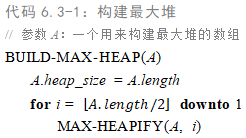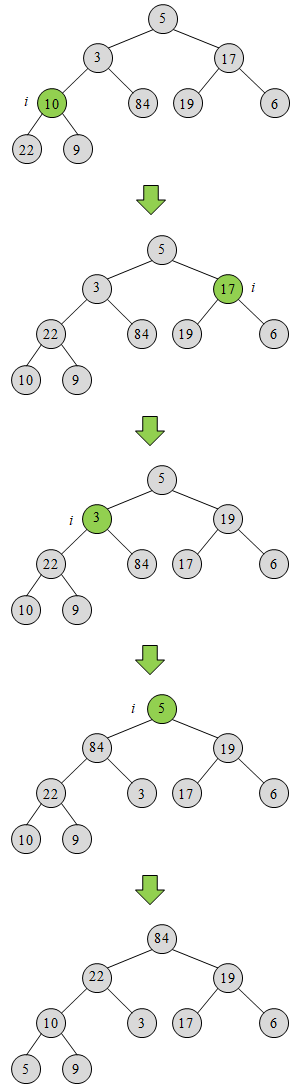# 算法导论 — 6.3 建堆

### 笔记BUILD-MAX-HEAP的运行时间为$O(n)$。这一结果的推导过程可以参考书本上的描述，这里不做说明。
下图给出了一个构建最大堆的例子。### 练习

6.3-1 参照图6-3的方法，说明BUILD-MAX-HEAP在数组A = <5, 3, 17, 10, 84, 19, 6, 22, 9>上的操作过程。6.3-2 对于BUILD-MAX-HEAP中第2行的循环控制变量$i$来说，为什么我们要求它是从$⌊A.length/2⌋$到1递减，而不是从1到$⌊A.length/2⌋$递增呢？

因为调用MAX_HEAPIFY(A, i)的先决条件是，结点$i$的子树必须都已经满足最大堆条件。而节点$i$的子树中的结点的下标都比$i$要大，因此BUILD-MAX-HEAP中的循环控制变量$i$必须是递减的。

6.3-3 证明：对于任一包含$n$个元素的堆中，至多有$⌈n/2^{h+1}⌉$个高度为h的结点？

首先考虑叶结点，它们的高度$h = 0$，根据练习6.1-7的结论，含有$n$个元素的堆的叶子结点的个数为$n-⌊n/2⌋=⌈n/2⌉=⌈n/2^{0+1}⌉$。因此命题对$h = 0$是成立的。
下面把原始堆$H_0$中的叶结点去掉，剩下的元素仍然构成一个堆$H_1$，并且$H_1$中的叶结点就是$H_0$中高度为$1$的结点。堆$H_1$的大小为$⌊n/2⌋$，故$H_1$中的叶结点个数为$⌈⌊n/2⌋/2⌉≤⌈n/2^2 ⌉$。即原始堆$H_0$中高度为$1$的结点至多有$⌈n/2^2 ⌉=⌈n/2^{1+1}⌉$个。因此，命题对$h = 1$也是成立的。
下面把堆$H_1$中的叶结点去掉，剩下的元素也构成一个堆$H_2$，并且$H_2$中的叶结点就是$H_0$中高度为$2$的结点。堆$H_2$的大小为$⌊⌊n/2⌋/2⌋$（因为堆$H_1$的大小为$⌊n/2⌋$，根据练习6.1-7的结论，堆$H_1$中的非叶结点个数为$⌊⌊n/2⌋/2⌋$）。堆$H_2$中的叶子结点个数为$⌈⌊⌊n/2⌋/2⌋/2⌉≤⌈n/2^3⌉$。即原始堆$H_0$中高度为$2$的结点至多有$⌈n/2^3 ⌉=⌈n/2^{2+1}⌉$个。因此，命题对$h = 2$也是成立的。
… …
以此类推，在一个大小为$n$的堆中，高度为$h$的结点至多有$⌈n/2^{h+1}⌉$个。